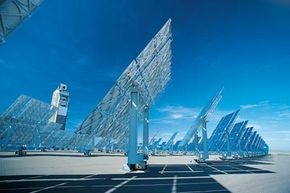# How many solar cells would I need in order to provide all of the electricity that my house needs?Location is crucial in determining how many solar cells are necessary to power a house. Digital Vision/Getty Images

If you have read the HSW article entitled How Solar Yard Lights Work, then you can get a feeling for how much power a solar cell can produce. The solar panel shown in that article contains 4 cells, and each of them can produce 0.45 volts and 100 milliamps, or 45 milliwatts. Each cell measures 2 inches by 0.5 inches. In other words, with these solar cells you can generate 45 milliwatts in one square inch (6.45 square cm). For the sake of discussion, let's assume that a panel can generate 70 milliwatts per square inch.

To calculate how many square inches of solar panel you need for a house, you need to know:

• How much power the house consumes on average.
• Where the house is located (so you can calculate mean solar days, average rainfall, etc.). This question is impossible to answer unless you have a specific location in mind. We'll assume that on an average day the solar panels generate their maximum power for 5 hours.

The first question is actually pretty interesting, so let's work on it.

A "typical home" in America can use either electricity or gas to provide heat -- heat for the house, the hot water, the clothes dryer and the stove/oven. If you were to power a house with solar electricity, you would certainly use gas appliances because solar electricity is so expensive. This means that what you would be powering with solar electricity are things like the refrigerator, the lights, the compute­r, the TV, stereo equipment, motors in things like furnace fans and the washer, etc. Let's say that all of those things average out to 600 watts on average. Over the course of 24 hours, you need 600 watts * 24 hours = 14,400 watt-hours per day.

­From our calculations and assumptions abo­ve, we know that a solar panel can generate 70 milliwatts per square inch * 5 hours = 350 milliwatt hours per day. Therefore you need about 41,000 square inches of solar panel for the house. That's a solar panel that measures about 285 square feet (about 26 square meters). That would cost around \$16,000 right now. Then, because the sun only shines part of the time, you would need to purchase a battery bank, an inverter, etc., and that often doubles the cost of the installation.

If you want to have a small room air conditioner in your bedroom, double everything.

Because solar electricity is so expensive, you would normally go to great lengths to reduce your electricity consumption. Instead of a desktop computer and a monitor you would use a laptop computer. You would use fluorescent lights instead of incandescent. You would use a small B&W TV instead of a large color set. You would get a small, extremely efficient refrigerator­. By doing these things you might be able to reduce your average power consumption to 100 watts. This would cut the size of your solar panel and its cost by a factor of 6, and this might bring it into the realm of possibility.

The thing to remember, however, is that 100 watts per hour purchased from the power grid would only cost about 24 cents a day right now, or \$91 a year. That's why you don't see many solar houses unless they are in very remote locations. When it only costs about \$100 a year to purchase power from the grid, it is hard to justify spending thousands of dollars on a solar system.

­